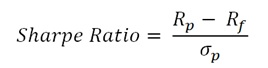We are all experts in some things! Experts of Mutual Funds enter here.

While evaluating an investment option, we generally tend to see the returns generated by the investment option and the risk involved in investing as two separate things. There is a way in which both returns and risks involved in a particular investment can be clubbed to arrive at a concept called “Risk Adjusted Returns”.

Risk adjusted returns are the excess returns generated by fund for the every unit of risk associated with it.Broadly we could use Sharpe ratio and Treynor ratio to measure the risk adjusted returns.

## 1. Sharpe Ratio:

In arriving at the risk adjusted returns, Sharpe ratio considers the returns generated by the fund and the risk free rate (Government security/Treasure bills rates are considered as risk free) to arrive at the excess rate.If the fund/portfolio returns are 18% and the risk free rate is 8%, the excess return generated by the fund is 10%. The fund has generated 10% over and above the risk free rate, which is nothing but the “Risk premium” of the fund. Standard deviation is used to assess how much risk is involved in generating the excess returns. If the standard deviation of the fund is 4, then the Sharpe ratio of the fund is 2.50.

Fund Sharpe Ratio Standard Deviation
Fund B 0.64 16.86
Fund D 0.38 17.98
Fund F 0.68 14.84
Fund H 0.27 18.74
Fund R 0.39 20.02
Fund T 0.28 16.75
Fund U 0.54 14.13

When comparing the Sharpe ratio, higher the ratios better the fund. In the above mentioned illustration, Fund F and B have the higher Sharpe ratio in the fund category, which are better funds. The reason for better Sharpe ratio is portfolio risk (Standard Deviation) of the fund is among the lowest.

## 2. Treynor Ratio:

Both Sharpe and Treynor ratio measures risk adjusted return. Treynor ratio uses mutual fund/portfolio beta while calculating risk adjusted returns, where as Sharpe ratio uses Standard deviation.The interpretation of the ratio would remain the same;what is the risk taken, to generate the excess returns.A fund with higher the ratio in the peer group is considered to be better fund in the category.

Jensen’s Alpha:
Alpha is another risk adjusted returns measurement tool which measures the fund manager’s outperformance. “Alpha gives the excess returns over the expected rate of return”. The objective of the investment is to generate optimum returns with least possible risk. A fund/portfolio with higher Alpha among its peers would be the fund which generates higher return with least risk.

Fund Alpha = Fund’s Actual returns – Expected rate of returns

Fund Alpha
Fund B 8.91
Fund D 4.77
Fund F 8.41
Fund H 2.95
Fund R 5.49
Fund T 2.68
Fund U 5.93

As per the illustration given above, Fund B and Fund F have higher Alpha in the category. They generate higher returns for the limited risk taken by the fund. Both of these funds could be ideal choices for an investor to have in his portfolio.

Expected rate of returns is arrived at by using the Capital Asset Pricing Model (CAPM). The CAPM uses market risk i.e., Fund Beta to compute the expected rate of returns (ER).

ER =Rf+β*(Rm-Rf)
ER = Expected rate of returns
Rf= Risk free rate
Β = Fund/portfolio beta
Rm= Marketreturns

The above table is for illustration purpose only & shall not be construed as indicative yields/returns of any of the Schemes of Canara Robeco Mutual Fund. Past performance may or may not be sustained in the future.

Take the Quiz

×